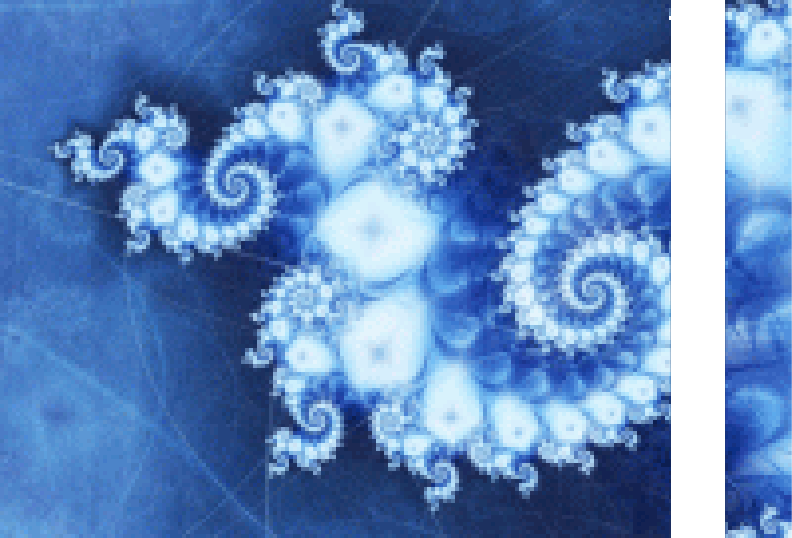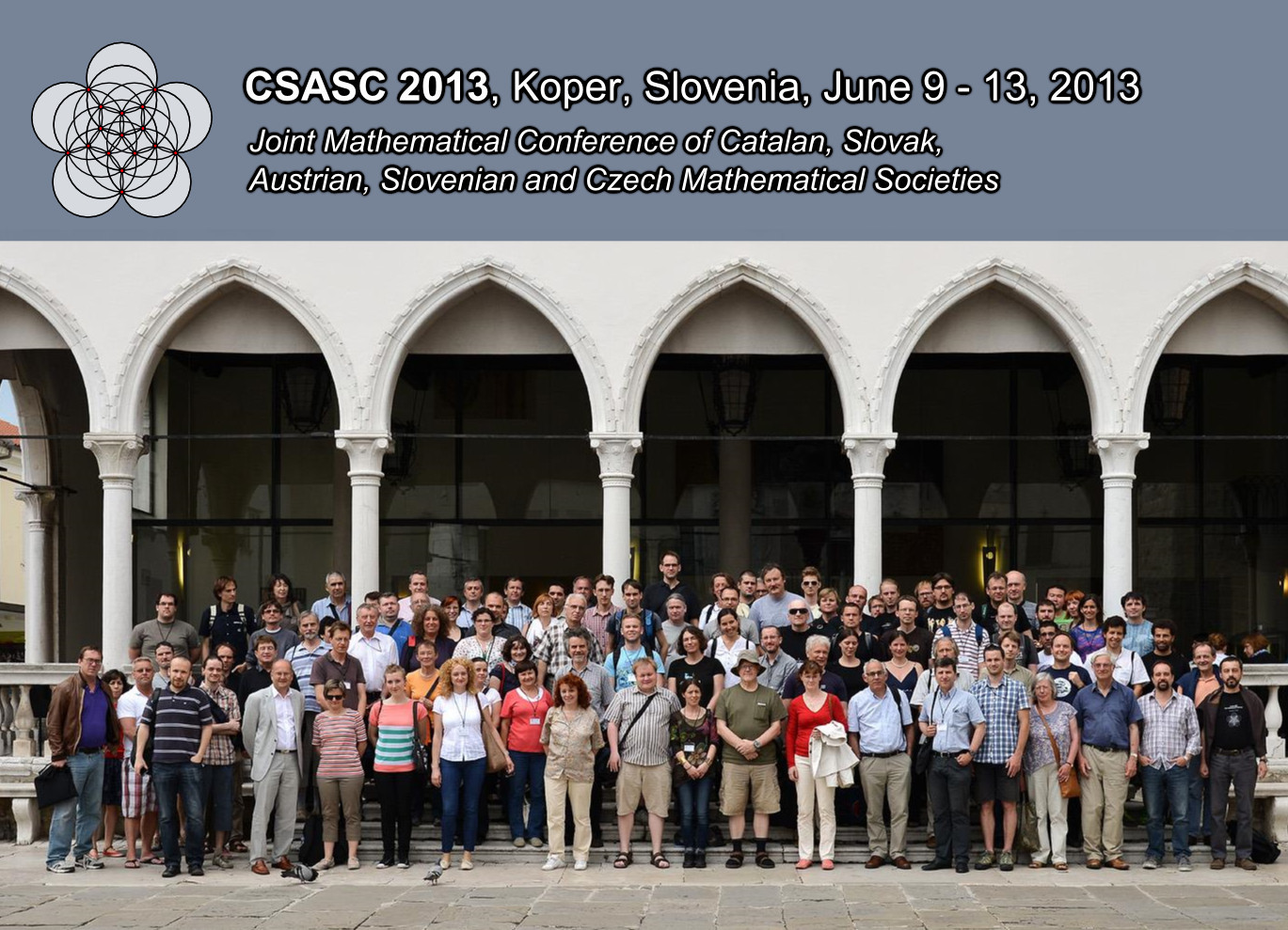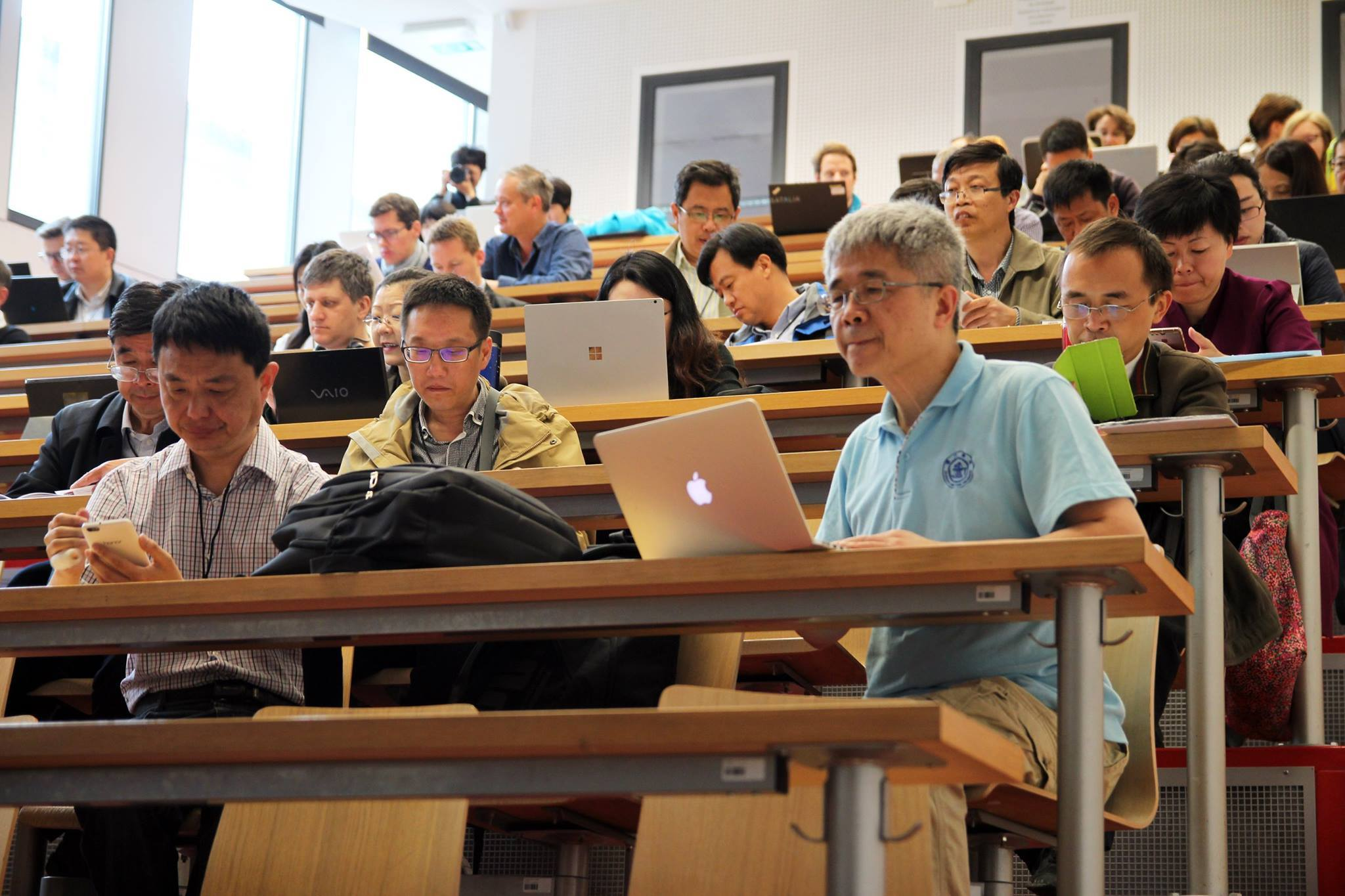Univerza na Primorskem Fakulteta za matematiko, naravoslovje in informacijske tehnologijeSI | EN

# Graph Theory Semester - Roman Nedela

natisni

Graphs and their coverings

How to show that each toroidal map is 7-colourable? How to describe the Hoffman-Singleton graph in one-line? How to prove that there are infinitely many 5-transitive graphs, or that there are regular graphs of arbitrarily large girth? How to prove that every Cayley graph based on a solvable group admits a nowhere-zero 4-flow? These essentially different problems have one point in common. All they can be solved using the powerful technique of graph coverings.

Graph coverings are homomorphism between graphs that are locally bijective. It is well-known, that a graph covering $G\to H$ can be reconstructed from the image $H$ employing voltage assignments defined on the arcs of $H$ and taking values in a group. If the graph $H$ is a one-vertex graph and the covering is regular, then the reconstruction of $G$ from $H$ is known as the construction of a Cayley graph. Birth of the theory of voltage assignments and dual theory of current graphs is closely related to the Ringel's solution of the Heawood map colouring problem. Firstly, it turned out that the problem of determining the chromatic number of a closed surface of Euler characteristic $\leq 0$  is equivalent to the problem of determining of genera of the complete graphs $K_n$, $n\geq 5$. To construct a genus embedding of a complete graph it is sufficient to construct a triangulation, or almost a triangulation, whose underlying graph is a complete graph. In this stage, the theory of graph coverings was used. Namely, starting from a small embedding of a graph using proper voltage assignments a genus embedding of $K_n$ is reconstructed. Further development of the theory was boosted by the monograph by Gross and Tucker (1987). Another, parallel line of research employing graph coverings, was an investigation of highly symmetrical graphs and maps. In particular, the theory can be used as a powerful tool to solve the difficult lifting automorphism problem for many interesting instances, or at least to reduce it to a problem of linear algebra. The theory of graph coverings is nowadays established as an integral part of graph theory, offering a powerful tool to construct graphs
sharing prescribed properties.

In the proposed course we first introduce basic concepts and results. Later we shall deal with applications including constructions of highly symmetrical graphs and maps, enumeration problems, existence of regular graphs with large girth, the degree-diameter problem, flow problems and others.

Lecturer: Roman Nedela, Matej Bel University, Slovakia